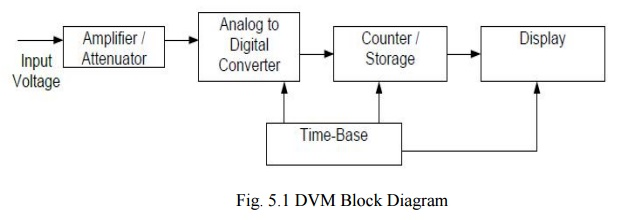Home | | Electrical Engineering and Instrumentation | Digital Voltmeter (DVM)

# Digital Voltmeter (DVM)

(i) Ramp type Digital Voltmeter (ii) Dual slope integrating type Digital Voltmeter

Digital Voltmeter (DVM)

It is a device used for measuring the magnitude of DC voltages. AC voltages can be measured after rectification and conversion to DC forms. DC/AC currents can be measured by passing them through a known resistance (internally or externally connected) and determining the voltage developed across the resistance (V=IxR). The result of the measurement is displayed on a digital readout in numeric form as in the case of the counters. Most DVMs use the principle of time period measurement. Hence, the voltage is converted into a time interval â€śtâ€ť first. No frequency division is involved. Input range selection automatically changes the position of the decimal point on the display. The unit of measure is also highlighted in most devices to simplify the reading and annotation.

The block diagram shown below illustrates the principle of operation of a digital voltmeter. It is composed of an amplifier/attenuator, an analog to digital converter, storage, display and timing circuits. There is also a power supply to provide the electrical power to run electronic components. The circuit components except the analog to digital converter circuits are similar to the ones used in electronic counters. The input range selection can be manually switched between ranges to get most accurate reading or it can be auto ranging that switches between ranges automatically for best reading.## (i) Ramp type Digital Voltmeter

Functional block diagram of a positive ramp type DVM is shown below. It has two major sections as the voltage to time conversion unit and time measurement unit. The conversion unit has a ramp generator that operates under the control of the sample rate oscillator, two comparators and a gate control circuitry. The internally generated ramp voltage is applied to two comparators. The first comparator compares the ramp voltage into the input signal and produces a pulse output as the coincidence is achieved (as the ramp voltage becomes larger than the input voltage). The second comparator compares the ramp to the ground voltage (0 volt) and produces an output pulse at the coincidence. The input voltage to the first comparator must be between Vm. The ranging and attenuation section scales the DC input voltage so that it will be within the dynamic range. The decimal point in the output display automatically positioned by the ranging circuits.Block Diagram of Ramp Type (Single Slope) DVM

## (ii) Dual slope integrating type Digital Voltmeter

The ramp type DVM (single slope) is very simple yet has several drawbacks. T he major limitation is the sensitivity of the output to system components and clock. The dual slope techniques eliminate the sensitivities and hence the mostly implemented approach in DVMs. Th e operation of the integrator and its output waveform are shown below.The integrator works in two phases as charging and discharging. In phase-1, the switch connects the input of the integrator to the unknown input voltage (Vin) for a predetermined time T and the integrator capacitor C charges through the input resistor R.

The block diagram of the dual- slope type DVM is below. The figure illustrates the effects of the input voltage on charging and di scharging phases of the converter. The total conversion time is the sum of the charging and discharging times. Yet, only the discharging ti me is used for the measurement and it is independent of the system components R and C, and the clock frequency.Study Material, Lecturing Notes, Assignment, Reference, Wiki description explanation, brief detail
Electrical Engineering and Instrumentation : Analog and Digital Instruments : Digital Voltmeter (DVM) |

Related Topics